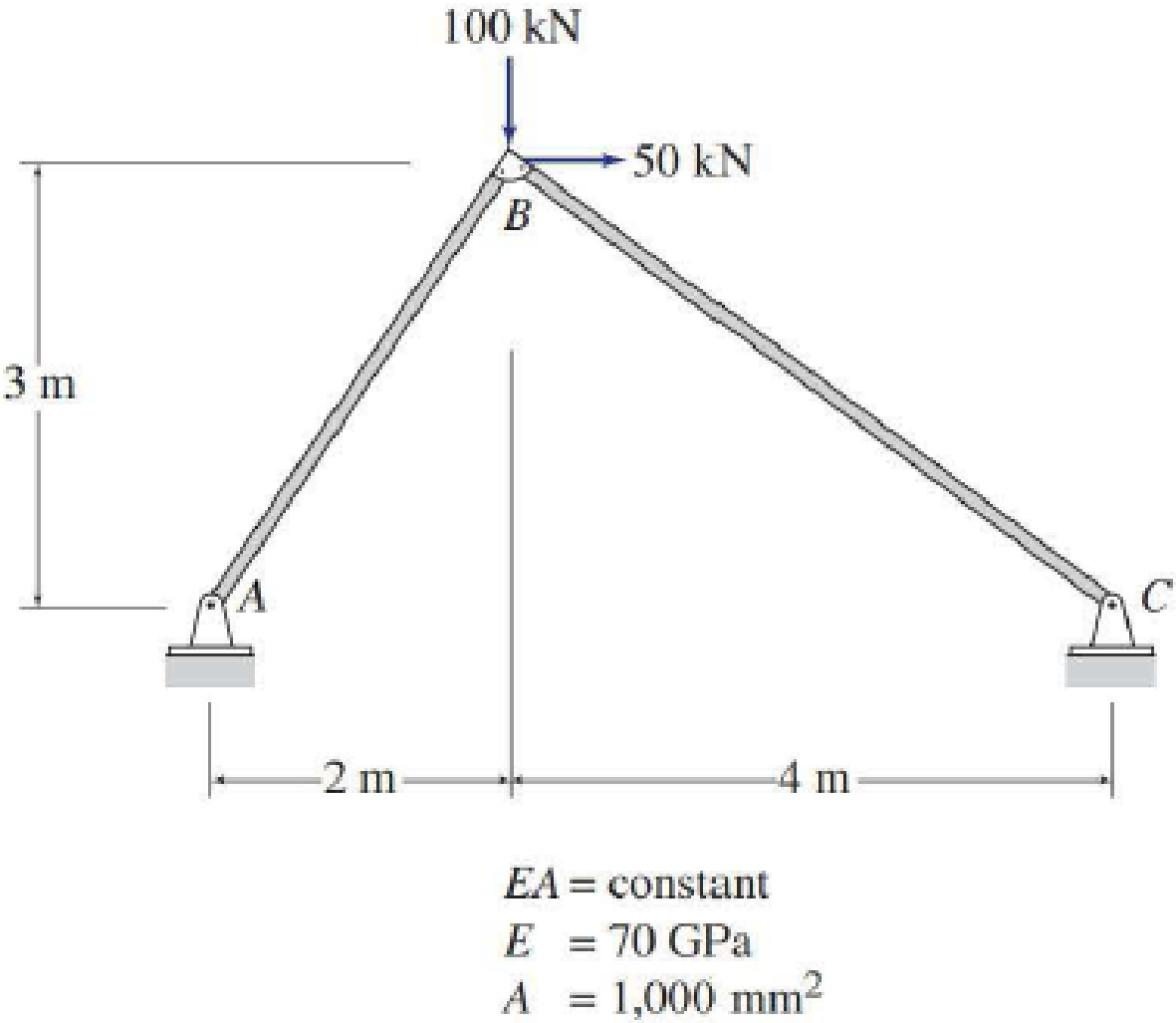# 7.51 through 7.55 Use Castigliano’s second theorem to determine the horizontal and vertical components of the deflection at joint B of the trusses shown in Figs. P7.1–P7.5. FIG. P7.2, P7.52

#### Solutions

Chapter
Section
Chapter 7, Problem 52P
Textbook Problem
59 views

## 7.51 through 7.55 Use Castigliano’s second theorem to determine the horizontal and vertical components of the deflection at joint B of the trusses shown in Figs. P7.1–P7.5.FIG. P7.2, P7.52To determine

Calculate the horizontal deflection and vertical deflection at joint B of the truss.

### Explanation of Solution

Given information:

The truss is given in the Figure.

The value of E is 70 GPa and the value of A is 1,000mm2.

Apply the sign conventions for calculating reactions, forces and moments using the three equations of equilibrium as shown below.

• For summation of forces along x-direction is equal to zero (Fx=0), consider the forces acting towards right side as positive (+) and the forces acting towards left side as negative ().
• For summation of forces along y-direction is equal to zero (Fy=0), consider the upward force as positive (+) and the downward force as negative ().
• For summation of moment about a point is equal to zero (Matapoint=0), consider the clockwise moment as negative and the counter clockwise moment as positive.

Method of joints:

The negative value of force in any member indicates compression (C) and the positive value of force in any member indicates tension (T).

Condition for zero force members:

1. 1. If only two non-collinear members are connected to a joint that has no external loads or reactions applied to it, then the force in both the members is zero.
2. 2. If three members, two of which are collinear are connected to a joint that has no external loads or reactions applied to it, then the force in non-collinear member is zero.

Calculation:

Let apply a horizontal load P1 at joint B in the horizontal direction to find the horizontal deflection.

The value of load P1 is 50 kN.

Let Ax and Ay be the horizontal and vertical reactions at the hinged support A.

Let Cx and Cy be the horizontal and vertical reactions at the hinged support C.

Sketch the resultant truss diagram for horizontal load P1 at joint B as shown in Figure 1.

Find the reactions at the supports using equilibrium equations:

Summation of moments about C is equal to 0.

MC=0Ay(6)P1(3)=0Ay=0.5P1

Summation of forces along y-direction is equal to 0.

+Fy=0Ay+Cy=00.5P1+Cy=0Cy=0.5P1

Consider AB,

Summation of moments about B is equal to 0.

MB=0Ay(2)Ax(3)=0(0.5P1)(2)Ax(3)=0Ax=0.333P1

Summation of forces along x-direction is equal to 0.

+Fx=0AxCx+P1=00.333P1Cx+P1=0Cx=0.667P1

Apply equilibrium equation to the joint A:

Summation of forces along y-direction is equal to 0.

0.5P1+FABsin56.31°=0FAB=0.601P1

Apply equilibrium equation to the joint C:

Summation of forces along y-direction is equal to 0.

0.5P1+FBCsin36.87°=0FBC=0.833P1

Let apply a vertical load P2 at joint B in the vertical direction to find the vertical deflection.

The value of load P2 is 100 kN.

Sketch the resultant truss diagram for vertical load P2 at joint B as shown in Figure 2.

Find the reactions at the supports using equilibrium equations:

Summation of moments about C is equal to 0.

MC=0Ay(6)P2(4)=0Ay=0.667P2

Summation of forces along y-direction is equal to 0.

+Fy=0Ay+CyP2=00.667P2+CyP2=0Cy=0.333P2

Consider AB,

Summation of moments about B is equal to 0.

MB=0Ay(2)+Ax(3)=0(0.667P2)(2)+Ax(3)=0Ax=0.444P2

Summation of forces along x-direction is equal to 0.

+Fx=0AxCx=00.444P2Cx=0Cx=0.444P2

Apply equilibrium equation to the joint A:

Summation of forces along y-direction is equal to 0.

0.667P2+FABsin56.31°=0FAB=0.801P2

Apply equilibrium equation to the joint C:

Summation of forces along y-direction is equal to 0.

0.333P2+FBCsin36.87°=0FBC=0.566P2

The total force F in a member is equal to the sum of the force in the member due to horizontal load P1 and vertical load P2

### Still sussing out bartleby?

Check out a sample textbook solution.

See a sample solution

#### The Solution to Your Study Problems

Bartleby provides explanations to thousands of textbook problems written by our experts, many with advanced degrees!

Get Started

Find more solutions based on key concepts
What percentage of the U.S. electric power is generated using nuclear fission?

Engineering Fundamentals: An Introduction to Engineering (MindTap Course List)

What is the purpose of the leads crew of a lathe?

Precision Machining Technology (MindTap Course List)

When you take photos on a digital camera, the camera often generates a file name consisting of a generic prefix...

Enhanced Discovering Computers 2017 (Shelly Cashman Series) (MindTap Course List)

On which networking device do you configure VLANs?

Network+ Guide to Networks (MindTap Course List)

Will online transactions eventually replace person-to-person contact? Why or why not?

Systems Analysis and Design (Shelly Cashman Series) (MindTap Course List)

If your motherboard supports ECC DDR3 memory, can you substitute non-ECC DDR3 memory?

A+ Guide to Hardware (Standalone Book) (MindTap Course List)